## How to hide error values

In Excel table may occur some error values like #DIV/0, #N/A … But it does not necessarily mean an error in a formula. It could mean that there is some unfilled value or some value is not in table and so on. In this cases is better display nothing or some specific word or number instead of error value.  I have prepared table containing #DIV/0 values and two ways how to solve it. The first is using formula and the second is made by conditional formatting.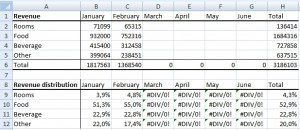The bottom table have simple formula for calculating percentage of items revenue. For example in B9 is formula =B2/B\$6. So, when cells in the top table is empty, result of formula is #DIV/0. Continue reading “How to hide error values” »

## How to quickly lock (protect) all of the cells containing formula

There are many values and formulas on the sheet. We want to protect all formulas against overwriting. Other cells must remain unlocked for entry. We don’t need to manually go through cell by cell.

This is a table with some formulas: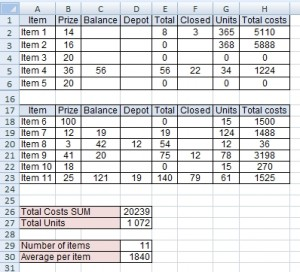Let’s see how to do that: Continue reading “How to quickly lock (protect) all of the cells containing formula” »

## Average with multiple conditions

There is problem with average for some specific values in a table. We need to make average of the items that meet several criteria. Following table represents data of some worldwide company. You can see branch offices in the cities and number of their new customers per each month and year.How to do average of specific branch offices for specific year? Continue reading “Average with multiple conditions” »

## Average with condition

There is a problem with average for some specific value in a table. Following table represents data of some worldwide company. You can see branch offices in the cities and number of their new customers per each month.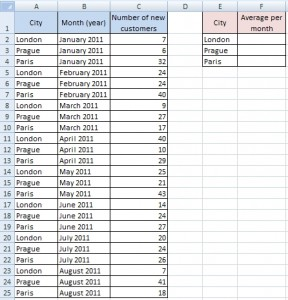How to do average for specific items (London, Prague and Paris)? Continue reading “Average with condition” »

## Address of the first empty cell in a row

In some cases it is useful to know the address of the first empty cell in a row. This is a way to do this without macros. Write the following formula and press CTRL+SHIFT+ENTER. Excel adds braces { } and saves it as array formula.

## Address of the first empty cell in a column

In some cases it is useful to know the address of the first empty cell in a column. This is a way to do this without macros. Write the following formula and press CTRL+SHIFT+ENTER. Excel adds braces { } and saves it as array formula.

```=ADDRESS(ROW(INDEX(firstCell:lastCell,
MATCH(TRUE,LEN(firstCell:lastCell)=0,0),1)),column number)```

## Running total for multiple items solved by array formula

There are some stuff dealers. In the following table is list of their sales. We need to know, how many sales they made from the beginning of the year. But there is a problem, we need to know sum to the date in each record for the dealer. For example: Jack Smith sold 1 piece on 2011/01/07 and then 3 pieces on 2011/01/19. So total to 2011/01/07 is 1, but total to 2011/01/19 is 4 (1+3).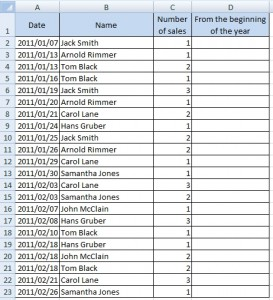Question is how to create running total for each dealer in the table. Continue reading “Running total for multiple items solved by array formula” »

## Number of rows that (not) contain a specific value

I prepared two ways to solve this problem. The first uses values in a new column and the second uses array formula. Both have their advantages.

## Example

Table in the picture below contains information about the success of students making 3 exams. Value “Yes” means he passed, value “No” means he did not pass. Student should have value “Yes” in each column to pass all exams.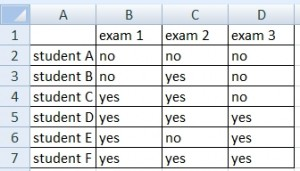How many students pass all of exams? The question is, how to count rows having value “Yes” in column B or C or D. Continue reading “Number of rows that (not) contain a specific value” »